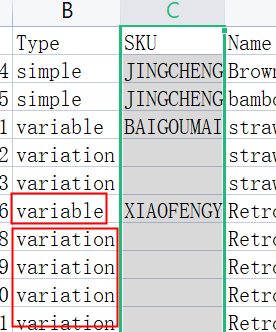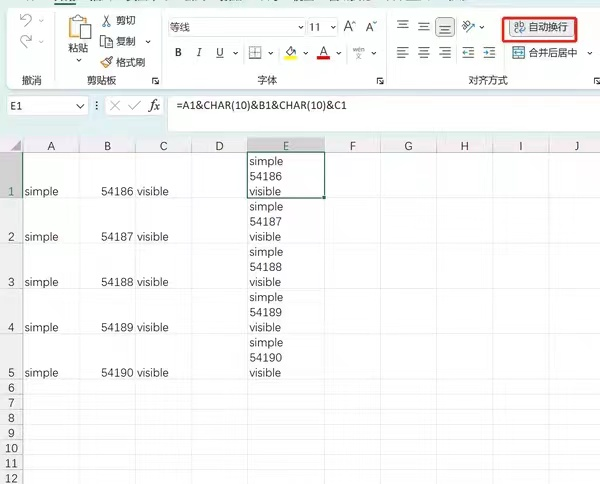# excel两列数据合并覆盖 | 我的SEO

excel两列数据合并覆盖

vlookup      和 =IF(A1="","",VLOOKUP("*"&A1&"*",B:B,1,0))

1：若要将两个Excel表格中相同的数据进行覆盖合并成新的表格，可以按照以下步骤操作：

打开Excel软件并将两个表格同时打开。

选中其中一个表格，复制表格中所有数据。

切换到另一个表格，将光标移动到要覆盖的位置，选择“编辑”->“粘贴特殊”->“数值”，然后点击“确定”按钮。

Excel会弹出一个“覆盖值”的对话框，选择“是”即可完成覆盖合并。

完成上述步骤后，您就可以得到一个新的表格，其中包含两个表格中相同数据的最新更新。if(前面的单元格编号 = "-","-","")

=if(j1<>"",j1,k1)   覆盖合并

=IF(AND(J1="",K1=""),"",IF(J1<>"",J1,K1))   覆盖合并

if(前面的单元格编号 = "-","-","")

=IF(AND(J1="",K1=""),"",IF(J1<>"",J1,K1))   覆盖合并

=IF(AND(A1="",B1=""),"",IF(A1<>"",A1,B1))A.B 列合并（自动换行）
=A1&CHAR(10)&B1&CHAR(10)&C1

11111